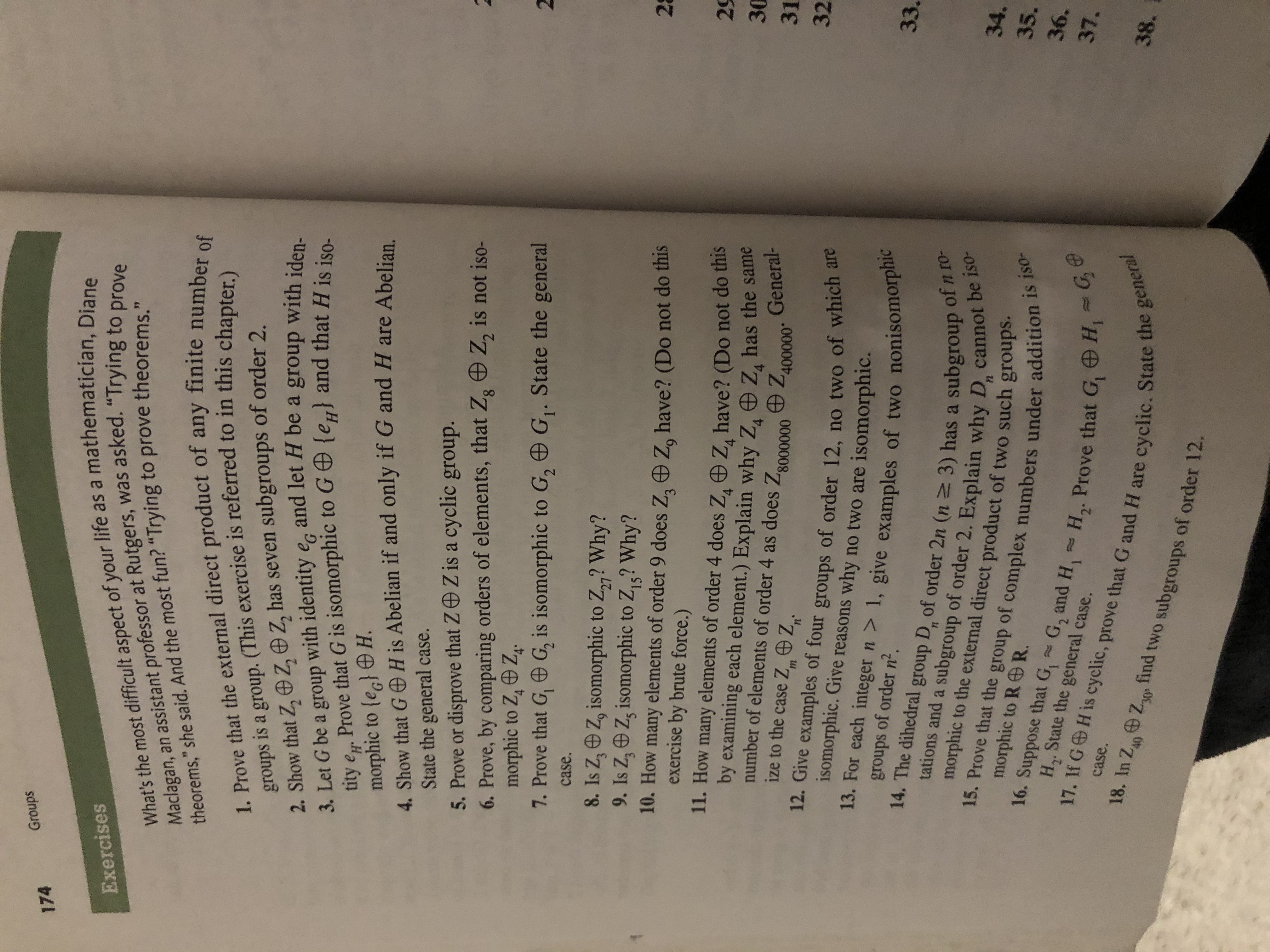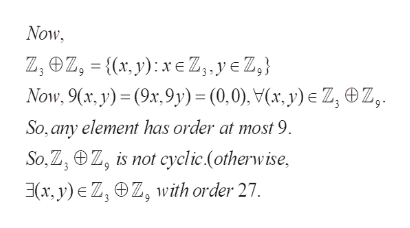# Groups174What's the most difficult aspect of your life as a mathematician, DianeMaclagan, an assistant professor at Rutgers, was asked. "Trying to provetheorems," she said. And the most fun? "Trying to prove theorems."Exercises1. Prove that the external direct product of any finite number ofsa group. (This exercise is referred to in this chapter.)2. Show that Z, Z, Z, has seven subgroups of order 23. Let G be a group with identity e and let H be a group with iden-tity e Prove that G is isomorphic to Ge} and that H is isomorphic to (eg) H.4. Show that G H is Abelian if and only if G and H are AbelianState the general case.5. Prove or disprove that Z Z is a cyclic group.6. Prove, by comparing orders of elements, that Zg Z, is not iso-morphic to Z, Z.7. Prove that G, G, is isomorphic to G, G. State the generalgroups284case.8. Is Z,Z, isomorphic to Z2? Why?9. Is Z,z, isomorphic to Z,5? Why?10. How many elements of order 9 does Z Z, have? (Do not do thisexercise by brute force.)11. How many elements of order 4 does Z, Z, have? (Do not do thisby examining each element.) Explain why Z Znumber of elements of order 4 as does Zs00000 Z400000ize to the case Z Z.2930has the sameGeneral-313212. Give examples of four groups of order 12, no two of which areisomorphic. Give reasons why no two are isomorphic.13. For each integer n > 1, give examples of two nonisomorphic14. The dihedral group D, of order 2n (n 3) has a subgroup of n ro-morphic to the external direct product of two such groups.of order n2.groups33.tations and a subgroup of order 2. Explain why D, cannot be iso-15. Prove that the group of complex numbers under addition is iso-16. Suppose that G, G2 and H, H2. Prove that G, H G34.35.morphic to RR.36.H,. State the general case.17. If GH is cyclic, prove that G and H are37.case.18. In Z4 Z38.cyclic. State the generafind two subgroups of order 12.30

Question
1 views

8help_outlineImage TranscriptioncloseGroups 174 What's the most difficult aspect of your life as a mathematician, Diane Maclagan, an assistant professor at Rutgers, was asked. "Trying to prove theorems," she said. And the most fun? "Trying to prove theorems." Exercises 1. Prove that the external direct product of any finite number of sa group. (This exercise is referred to in this chapter.) 2. Show that Z, Z, Z, has seven subgroups of order 2 3. Let G be a group with identity e and let H be a group with iden- tity e Prove that G is isomorphic to Ge} and that H is iso morphic to (eg) H. 4. Show that G H is Abelian if and only if G and H are Abelian State the general case. 5. Prove or disprove that Z Z is a cyclic group. 6. Prove, by comparing orders of elements, that Zg Z, is not iso- morphic to Z, Z. 7. Prove that G, G, is isomorphic to G, G. State the general groups 2 8 4 case. 8. Is Z,Z, isomorphic to Z2? Why? 9. Is Z,z, isomorphic to Z,5? Why? 10. How many elements of order 9 does Z Z, have? (Do not do this exercise by brute force.) 11. How many elements of order 4 does Z, Z, have? (Do not do this by examining each element.) Explain why Z Z number of elements of order 4 as does Zs00000 Z400000 ize to the case Z Z. 29 30 has the same General- 31 32 12. Give examples of four groups of order 12, no two of which are isomorphic. Give reasons why no two are isomorphic. 13. For each integer n > 1, give examples of two nonisomorphic 14. The dihedral group D, of order 2n (n 3) has a subgroup of n ro- morphic to the external direct product of two such groups. of order n2. groups 33. tations and a subgroup of order 2. Explain why D, cannot be iso- 15. Prove that the group of complex numbers under addition is iso- 16. Suppose that G, G2 and H, H2. Prove that G, H G 34. 35. morphic to R R. 36. H,. State the general case. 17. If GH is cyclic, prove that G and H are 37. case. 18. In Z4 Z 38. cyclic. State the genera find two subgroups of order 12. 30 fullscreen
check_circle

Step 1

To decide if the first group (the direct sum) is isomorpbic to Z27,

Step 2

Description of the groups whose isomorphism is under question. The question arises because both (the direct sum as well as Z27 contain the same number of elements:27). We will show that these two groups are NOT isomorphic

Step 3

First observe that the direct su...help_outlineImage TranscriptioncloseNow, Z, ez,= {(x, y): xe Z, , ye Z,} Now, 9(x, y) (9x,9y) = (0,0), V(x, y) e Z, OZ, So, any element has order at most 9 So,Z, z, is not cyclic.(otherwise (x, y) Z, Z, with order 27. fullscreen

### Want to see the full answer?

See Solution

#### Want to see this answer and more?

Solutions are written by subject experts who are available 24/7. Questions are typically answered within 1 hour.*

See Solution
*Response times may vary by subject and question.
Tagged in

### Math